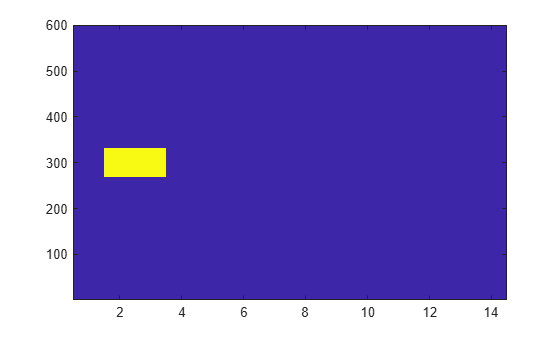# ltePSSSIndices

PSSS resource element indices

## Syntax

``ind = ltePSSSIndices(ue)``
``ind = ltePSSSIndices(ue,opts)``

## Description

example

````ind = ltePSSSIndices(ue)` returns a 124-by-1 complex column vector of resource element (RE) indices for the primary sidelink synchronization signal (PSSS) values for user equipment settings in the `ue` structure. By default, the indices are returned in one-based linear indexing form. You can use this form to directly index elements of a matrix representing the subframe resource grid for antenna port 1020. For more information, see Primary Sidelink Synchronization Signal Indexing.```

example

````ind = ltePSSSIndices(ue,opts)` formats the returned indices using options specified by `opts`.```

## Examples

collapse all

Generate PSSS values and indices. Write the values into the PSSS resource elements in a synchronization subframe (normal cyclic prefix) and display an image of their locations.

Create a user equipment settings structure and a resource grid that has a 10 MHz bandwidth and normal cyclic prefix.

```ue.NSLRB = 50; ue.CyclicPrefixSL = 'Normal'; ue.NSLID = 1; subframe = lteSLResourceGrid(ue);```

Generate PSSS indices and display the first five indices. Load the PSSS symbols into the resource grid. Display an image showing the PSSS symbol locations.

```psss_indices = ltePSSSIndices(ue); psss_indices(1:5)```
```ans = 5x1 uint32 column vector 870 871 872 873 874 ```
```subframe(psss_indices) = ltePSSS(ue); imagesc(100*abs(subframe)); axis xy;```Generate PSSS indices using zero-based indexing style. Compare these indices to one-based indices.

Create a user equipment settings structure and a resource grid that has a 10 MHz bandwidth and normal cyclic prefix.

```ue.NSLRB = 50; ue.CyclicPrefixSL = 'Normal'; ue.NSLID = 1; subframe = lteSLResourceGrid(ue);```

Generate PSSS zero-based indices and view the first five indices.

```psss_indices = ltePSSSIndices(ue,'0based'); psss_indices_size = size(psss_indices)```
```psss_indices_size = 1×2 124 1 ```
`psss_indices(1:5)`
```ans = 5x1 uint32 column vector 869 870 871 872 873 ```

Generate PSSS one-based indices and view the first five indices.

```psss_indices = ltePSSSIndices(ue,'1based'); psss_indices_size = size(psss_indices)```
```psss_indices_size = 1×2 124 1 ```
`psss_indices(1:5)`
```ans = 5x1 uint32 column vector 870 871 872 873 874 ```

For zero-based indexing, the first assigned index is one lower than the one-based indexing style.

## Input Arguments

collapse all

UE-specific settings, specified as a structure containing these parameter fields:

Number of sidelink resource blocks, specified as an integer scalar from 6 to 110.

Example: `6`, which corresponds to a channel bandwidth of 1.4 MHz.

Data Types: `double`

Cyclic prefix length, specified as `'Normal'` or `'Extended'`.

Data Types: `char` | `string`

Output format options for resource element indices, specified as a character vector, cell array of character vectors, or string array. For convenience, you can specify several options as a single character vector or string scalar by a space-separated list of values placed inside the quotes. Values for `opts` when specified as a character vector include (use double quotes for string) :

Category Options Description

Indexing style

`'ind'` (default)

The returned indices are in linear index style.

`'sub'`

The returned indices are in `[subcarrier,symbol,port]` subscript row style.

Index base

`'1based'` (default)

The returned indices are one-based.

`'0based'`

The returned indices are zero-based.

Example: `'ind 1based'`, `"ind 1based"`, `{'ind','1based'}`, or `["ind","1based"]` specify the same formatting options.

Data Types: `char` | `string` | `cell`

## Output Arguments

collapse all

PSSS resource element indices, returned as an integer column vector or a three-column integer matrix. This output is generated using the UE-settings structure, `ue`. For more information, see Primary Sidelink Synchronization Signal Indexing.

collapse all

### Primary Sidelink Synchronization Signal Indexing

Use the indexing function, `ltePSSSIndices`, and the corresponding sequence function, `ltePSSS`, to populate the resource grid for the desired subframe number. The PSSS values are output by `ltePSSS`, ordered as they should be mapped, applying frequency-first mapping into the resource elements of the adjacent symbols using `ltePSSSIndices`. When indexing is zero-based, the SC-FDMA symbols used are {1,2} for normal cyclic prefix and {0, 1} for extended cyclic prefix.

### Note

The indicated symbol indices are based on TS 36.211, Section 9.7. However to align with the LTE Toolbox™ subframe orientation, these indices are expanded from symbol index per slot to symbol index per subframe.

For more information on mapping symbols to the resource element grid, see Resource Grid Indexing.

The primary sidelink synchronization signal (PSSS) is transmitted in the central 62 resource elements of two adjacent SC-FDMA symbols in a synchronization subframe. The same sequence of 62 complex values is repeated in each of the symbols, resulting in a 124-by-1 element vector returned by the `ltePSSS` function. The values of this sequence are ordered as they should be mapped into the resource elements of the adjacent symbols using `ltePSSSIndices`. If a terminal is transmitting PSSS, then the PSSS should be sent every 40 ms with the exact subframe dependent on the RRC signaled subframe number offset (syncOffsetIndicator-r12).
The PSSS is sent on antenna port 1020, along with the secondary sidelink synchronization signal (SSSS). A synchronization subframe also contains the PSBCH, which is sent on antenna port 1010. The transmission power of the PSSS symbols should be the same as the PSBCH therefore they should be scaled by $\sqrt{72}{62}}$ in a subframe. No PSCCH or PSSCH transmission will occur in a sidelink subframe configured for synchronization purposes.
As specified in TS 36.211, Section 9.7, the PSSS identity assignment depends on the network coverage. The set of all ${N}_{\text{ID}}^{\text{SL}}$ is divided into two sets, id_net {0, ..., 167} and id_oon {168, ..., 335}, which are used by terminals that are in-network and out-of-network coverage, respectively. The sidelink physical layer cell identity number, ${N}_{\text{ID}}^{\text{SL}}$, corresponds to the `ltePSSS` input UE settings structure field `ue`.`NSLID`. Within each set, all identities result in the same PSSS. For an in-network terminal, the `ue`.`NSLID` value corresponds to the RRC sidelink synchronization signal identity (slssid-r12) associated with the cell.